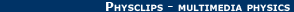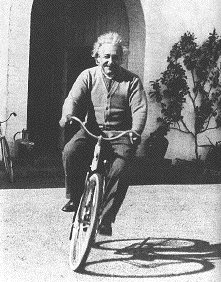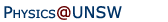Relativity in brief... or in detail..

## Inertial frames, Newtonian mechanics and why the laws are the same in the train and on the platform

### Inertial frames

Newton's laws work — sometimes. At other times, they appear not to work. Frames of reference in which Newton's laws of motion are observed are called Inertial Frames. For instance, the surface of the Earth is almost* an inertial frame. When we analyse the behaviour of objects with respect to the Earth, we nearly* always find that F = ma. Further, in the particular case where F = 0, objects maintain constant velocity.

* The 'almost' and 'nearly' are inserted above because of the Earth's own accelerated motion. (Travel in a straight line would make no difference, but rotation and orbital motion involve accelerations.) The daily rotation is quite slow: only one turn per day is 0.004 degree per second: this is why we cannot feel it. However, if we could observe motion over many minutes, we should notice that, for an object subject to negligible external forces, measurements made with respect to the laboratory frame show a very small acceleration. This acceleration is best considered as the acceleration of the laboratory with respect to the object: the result of the rotation of the laboratory, which turns with the Earth. The Earth is approximately, but not quite, an inertial frame. See the Foucault pendulum and coriolis forces for a more detailed discussion of this point.

Non inertial frames. In measurements made with respect to some other frames of reference, Newton's laws appear to be violated. For example, when the bus goes round a corner, a standing passenger who is not holding on seems to find himself accelerating sideways. If you try throwing and catching balls on a merry-go-round, you'll also observe some apparent violations of Newton's laws. Try this animation:

Zoe throws a ball from the centre of the a merry-go-round. To Zoe, turning clockwise with the merry-go-round (picture at left), the ball seems to curve to the left - it travels anti-clockwise. To an observer who is not rotating (e.g. someone in a tree above, picture at right, or to Jasper, sitting on the ground), the merry-go-round turns clockwise and the ball travels in a vertical plane (and thus a straight line, seen from above).

If you forget the outside world and refer everything to the frame of the merry-go-round, then you need to invent other fictitious forces which make moving objects turn. Newton's laws work in systems that are not spinning with respect to the distant galaxies. In frames of reference which spin, the 'extra forces' that have to be invoked to retain Newton's laws are fictitious forces called centrifugal forces and Coriolis forces.

 For a ball thrown on the surface of the earth, the earth hardly rotates during its flight (say 0.01 degree) so we do not notice the Coriolis force. For the sustained swinging of the Foucault pendulum, we could say that the Coriolis force makes it veer slightly to the left, and precess slowly anti-clockwise. Also in the Southern hemisphere, ocean currents and winds similarly tend to veer to the left: major circulations such as the South Pacific Current are anti-clockwise due to these Coriolis forces. Centrifugal forces are an example of imaginary forces invented to explain motion in a non-inertial frame. Why doesn't this man fall off his bicycle? An external observer would say that he is turning and therefore accelerating to the right (his left). If he could forget that he is in a non-inertial frame he might say that there is a mysterious 'centrifugal force' pushing him outwards, and he is leaning in against the 'force'. If he sat upright on the bicycle while turning, the 'centrifugal force' would seem to push him over sideways. If he closes his eyes, can he tell whether he is upright and travelling straight, or turning and leaning inwards on the corner? A negative answer to this question (or usually a more simple version involving linear acceleration) is the starting point for the General Theory of Relativity, which is Einstein's theory of gravitation and accelerated motion. But we're getting ahead of ourselves here!### More on the Galilean principle of relativity

This may be stated in a few different ways:
• A frame of reference that moves with constant velocity with respect to an inertial frame is also an inertial frame.
For example: Newton's laws work in the physics lab, which is fixed to the Earth. Therefore they also work in a train moving with constant velocity with respect to the Earth. (i.e. if you set up your experiments in a train moving smoothly, without turning, speeding up, slowing down or bumping, you will get the same results.)
One could also state it this way:
• If the laws of mechanics work in one frame of reference, then they also work in a frame of reference that moves with constant velocity with respect to the first frame.
(i.e. if your first frame is inertial, the second frame is inertial.) If you can juggle on the platform, you can juggle in the train, or even in a plane, even if a bystander sees you and the balls whizzing by at high speed.

### Why are the laws of physics the same in the train and on the platform?

The Galilean principle of relativity follows from a simple set of transformation equations and a few deceptively simple assumptions. These assumptions are so simple that most of us (though not Newton) would be likely to make them without thinking. For simplicity, let's confine all motion to the x direction. Suppose the train is moving with velocity v (in the x direction). To be concrete, let's suppose that the train is moving at 30 m/s. After one second, the train is 30 metres further on.

Now consider a passenger walking with constant velocity u = 1 m/s along the aisle, towards the front of the train. After one second, she is 1 metre further down the aisle. How far has she moved with respect to the platform? This looks like a simple question, but to answer it we need to make some assumptions about time and space. Let's assume, as Galileo and Newton quite reasonably did, that time is the same for all observers, provided that they have synchronised their clocks somewhere at some time, and that rulers have the same lengths for all observers. Okay, while I was carefully make my assumptions you beat me to it: the train has travelled 30 metres in the second, she is one metre further down the aisle, so she has travelled 31 metres with respect to the platform. She is travelling at v + u. In other words

In Galilean relativity, velocities add.
(We should point out that they add as vectors: you have to consider the angles if they are not in the same direction, but to a physicist adding vectors — things that have direction as well as magnitude — is easy enough and it's still addition.)
Now imagine that our passenger breaks into a run: she is accelerating. At one moment she is walking at 1 metre per second down the aisle, and a second later she is doing 3 metres per second, so her acceleration a in the train (we'll assume it constant) is 2 metres per second per second. What does the man on the platform see?

Well, making the same assumptions as before, he saw her first at 31 m/s, then a second later at 33 m/s, so he reckons that she accelerates at 2 metres per second per second also. This is a quite important point: observers in the train and on the platform disagree about the position and velocity of our runner, but not about her acceleration. Acceleration is the same whether observed from the train or from the station. (Remember that the train is travelling in a straight line at constant speed and direction — our argument only applies to uniform relative motion.)

So let's make more assumptions, no more unreasonable than those made earlier. We assume that both observers can agree on what forces (magnitude F) occur between her feet and the floor of the train, and that they agree on her mass m. Consequently, if F=ma is true for one of them, then F=ma is true for the other.

Under Galilean relativity, if Newton's laws work on the platform, they work on the uniformly moving train.
And that is why we are confident about the animation and the experiments discussed in Galilean relativity and Newton's laws. We have more to say about inertial frames later in this presentation. And more to say about those assumptions we made.

For more about Newtonian and Galilean mechanics, see Physclips: Mechanics with animations and film clips.Home | Summary | Quiz | Credits School of Physics - The University of New South Wales Sydney, Australia 2052 © School of Physics UNSW 2052 Disclaimer feedback